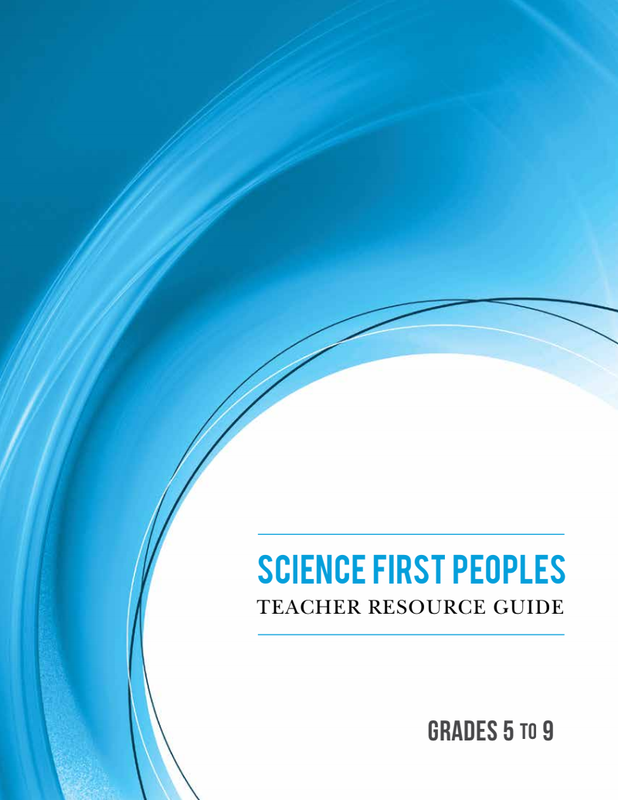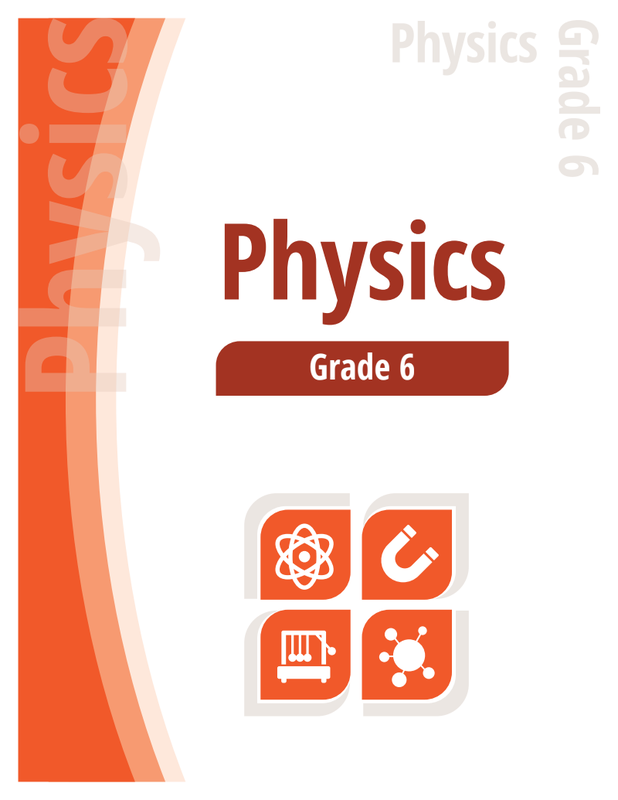Newton - Grade 6
Coast Meridian Elementary
Library Learning Commons
Roslyn Sundset
2018
Newton's 3 laws of motion
Relationship between force and motion
Science - First Peoples - Teachers guideForces and Motion Science Experiments | Steve Spangler Science Lab
A bunch of different experiments to do in the classroom
SCIENCE OF NHL HOCKEY: Newton’s Three Laws of Motion (Grades 5-8)
1.Students will design and carry out a demonstration of Newton’s first law of motion. 2.Students will design and carry out an investigation of Newton’s second law of motion. 3.Students will design and carry out a demonstration of the conservation of momentum and explain its relation to Newton’s third law of motion.
Lessons and Experiments from Science World
What happens when a bat hits a baseball? Why does a rolling ball eventually stop? How do we walk, jump or run? The answer lies in physics! In this topic, students get the chance to explore how Newton’s Laws of Motion explain the ways that things move.You won’t have to "force" your students to learn — they’ll push, pull, drop, roll, and fling their way through a variety of demonstrations and activities illustrating the different forces on everything around us.
force and motion, Newton’s Laws, gravity and inertia​
Force and Motion - There are 12 total matches.

Video list on force and motion